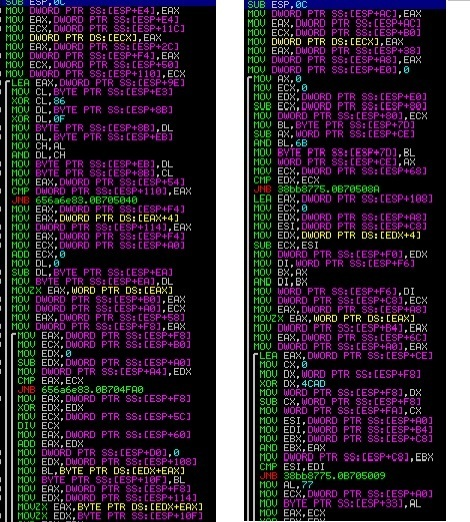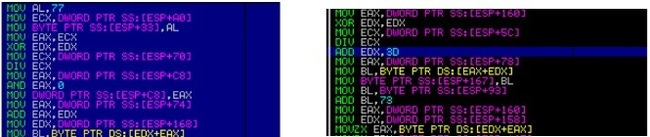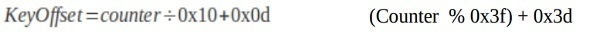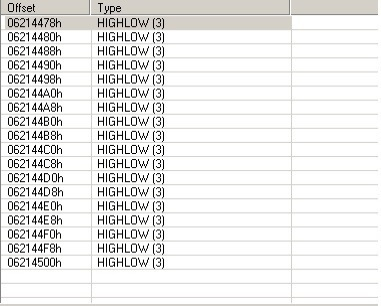# Understanding Neverquest Banking Trojan Polymorphic Engine

Neverquest packer uses polymorphic engine and junk code in its important subroutines. By using polymorphic engine to some extent static signature rules will fail. For example you can see the difference in between two main decoding subroutinesThe output of this subroutine is a LZ compressed buffer, which later on is submitted to APLIB decompression subroutine.

The main parts of this algorithm are*

1 :Key = variable length array of bytes rounded to 0 after list is exhausted

2 :Data Chunk Structure = variable length Array of structure defining length of block to be decoded +
pointer to that block

If we study the decoding algorithm we can fairly strip download the algorithm to a following simple
representation

``````
IF CHUNKSIZE > COUNTER : REPEAT
OUPUT := DATACHUNK – KEYBYTE_AT_VARIABLE_DISTANCE
``````

The main challenge with the algorithm is to get the base and the end of key . In some Case
it is 16 bytes and in some it is 256 bytes .

To understand how key-offset is taken we will two subroutine from two
different DLL’sThe Difference remains in key offset and key length . For the First one it is from 16 to 29 and for the
second one it is from 61 to 124, Which proves that the Key length and Key base are different in each casesNow comes the main challenge how to extract Key length and Key base. Now as we know that the
output of this decoder subroutine is LZ compressed buffer containing a valid PE image .
For a specific PE Image the PE stub , Headers and Sections would be same , so we will always get
the same type of data in first 100 bytes in the compressed buffer.

# Getting Key Length and Key base by brute-forcing #

In the decoding routine , which performs subtraction of key byte with data chunk . Subtraction operation is associative . We already know the value of Data Chunk and we have the key start location we can brute-force the key base and key length.

``````
void GetKeyLen(char *pbDataSection)
{
char LZNT_DEC[] =
"\xBE\xBA\x00\x4D\x5A\x90\x00\x03\x00\x00\x00\x82\x04\x00\x30\xFF\xFF\x00\x00\xB8\
x00\x38\x2D\x01\x00\x40\x04\x38\x19\x00\xF0\x00\x0C\x0E\x1F\x00\xBA\x0E\x00\xB4\x0
9\xCD\x21\xB8\x00\x01\x4C\xCD\x21\x54\x68\x69\x73\x00\x20\x70\x72\x6F\x67\x72\x61\
x6D\x00\x20\x63\x61\x6E\x6E\x6F\x74\x20\x00\x62\x65\x20\x72\x75\x6E\x20\x69\x00\x6
E\x20\x44\x4F\x53\x20\x6D\x6F\x80\x64\x65\x2E\x0D\x0D\x0A\x24\x04\x86\x00\xB2\x73\
xC1\xA4\xF6\x12\xAF\xF7\x41\x05\x03\xFF\x6A\x3A\xF7\xF7\x04\x0B\xF5\x11\x02\x0F\x3
C\xF7\xFD\x02\x0F\xAE\xF7\xA2\x41\x00\x07\x99\x64\x01\xF7\xFB\x02\x07\x34\x51\x02\
x27\x99\x64\x35\x04\x07\x32\x02\x07\x52\x18\x69\x63\x68\x01\x3B\x15\xB3\x50\x45\x0
0\x00\x00\x4C\x01\x05\x00\xA0\x49\x0E\x02\x52\x05\x13\xE0\x00\x02\x21\x0B\x01\x10\
x0A\x00\x00\x2A\x00\x06\x4A\x02\x00\x52\x00\x00\x01\xA0\x13\x80\x05\x10\x06\x84\x1
0\x63\x82\x05\x80\x0B\x05\x00\x01\x02\x08\x85\x03\x00\xC6\xB0\x00\x0A\x00\x9E\xB5\
xEC\x02\x01\x0F\x00\x0A\xCF\x00\x14\x81\x15\x86\x03\x03\x03\x20\x5C\x00\x2D\x80\x0
3\x20\x74\x58\x00\x00\x3C\x01\x0A\x60\x00\xD8\x00\x30\x37\x80\x18\x0D\x00\xA0\x83\
x22\x2E\x00\x10\x50\x00\x00\xA4\x18\x0F\x2E\x74\x65\xE4\x78\x74\x80\x03\x80\x29\x0
4\x48\x81\x75\x01\xFA\x45\x08\x00\x20\x40\x25\x62\x73\x73\xC2\x02\x90";
char KeyChar = 0;
char *offset = key;
int i = 3, j = 3;
printf("Data Section char == %x", *pbDataSection);
(unsigned char)KeyChar = (unsigned char)(*pbDataSection ­ LZNT_DEC);
printf("\nKey Char is = %c", (unsigned char)KeyChar);
while (1)
{
offset = CharSrch(offset, KeyChar, 512);
printf("\nOffset = %c", offset);
if (offset == NULL)
{
printf("[]... unknown error occured ...");
exit(­1);
}
if ( ( (unsigned char)LZNT_DEC == (unsigned char)(pbDataSection ­
offset) ) && ( ( (unsigned char)LZNT_DEC == (unsigned char)(pbDataSection
­ offset) ) ) ) // KeyLocation Found :)
{
printf("\nKey Location Found W00t = %s brute forcing Key length
now..", offset);
while (1)
{
printf("\nLZNT Chunk = %x, pbDataSection = %x, offset = %x,
Subtraction = %x", (unsigned char)LZNT_DEC[j] , (unsigned char)pbDataSection[j] ,
(unsigned char)offset[j], ((unsigned char)(pbDataSection[j] ­ offset[j])));
if (((unsigned char)LZNT_DEC[j] != (unsigned char)
(pbDataSection[j] ­ offset[j])) ) break;
i++;
j++;
}
printf("key length found = %d, %x != %x" , i,(unsigned
char)pbDataSection[j] , offset[j]);
key = offset;
keyLen = i;
iKeyFound = 1;
break;
}
offset++;
}
}
``````